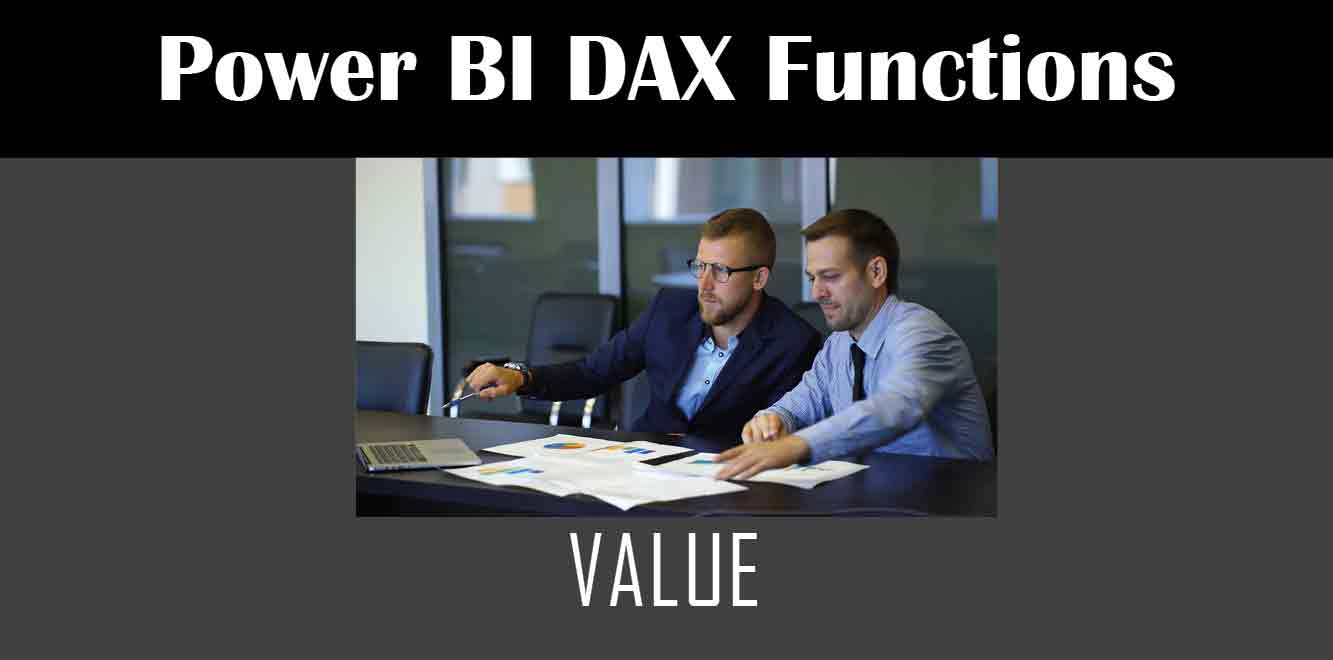# Value

## Y## What is the Power Fx Value Function?

The Power Fx Value function is used to convert text or other data types to a specific data type. For example, you can use it to convert a text string to a number or a date. The syntax for the Value function is as follows:

``` Value( value [, format ] ) ```

The value parameter is the text or data that you want to convert. The format parameter is optional and is used to specify the format of the data you want to convert.

Here are some examples of how to use the Value function:

``` Value("123") // returns the number 123 Value("01/01/2022", "mm/dd/yyyy") // returns the date January 1, 2022 ```

## Using the Power Fx Value Function in Power Apps

Now that we know what the Power Fx Value function is, let’s look at how we can use it in Power Apps.

### Converting Text to a Number

One of the most common use cases for the Value function is to convert text to a number. For example, let’s say you have a text input control in your Power App that allows users to enter a number. You can use the Value function to convert the text to a number so that you can perform calculations on it.

Here’s an example of how to use the Value function to convert text to a number:

``` Value(TextInput1.Text) ```

In this example, TextInput1 is the name of the text input control. The Text property of the control contains the text that the user entered. We pass this text to the Value function to convert it to a number.

### Converting Text to a Date

Another use case for the Value function is to convert text to a date. For example, let’s say you have a date input control in your Power App. You can use the Value function to convert the text to a date so that you can perform date calculations on it.

Here’s an example of how to use the Value function to convert text to a date:

``` Value(DateInput1.Text, "mm/dd/yyyy") ```

In this example, DateInput1 is the name of the date input control. The Text property of the control contains the text that the user entered. We pass this text to the Value function along with the format “mm/dd/yyyy” to convert it to a date.

### Converting Text to a Boolean

You can also use the Value function to convert text to a Boolean value. For example, let’s say you have a checkbox control in your Power App. You can use the Value function to convert the text “true” or “false” to a Boolean value.

Here’s an example of how to use the Value function to convert text to a Boolean:

``` Value(Checkbox1.Value) ```

In this example, Checkbox1 is the name of the checkbox control. The Value property of the control contains either “true” or “false” depending on whether the checkbox is checked or not. We pass this value to the Value function to convert it to a Boolean.

The Power Fx Value function is a powerful tool that allows you to convert text or other data types to a specific data type in Power Apps. By using this function, you can perform calculations or date manipulations on user input. We hope this article has provided you with a better understanding of how to use the Power Fx Value function in your Power Apps.